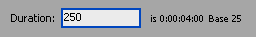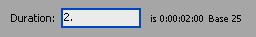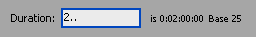Setting the Duration of a Composition

Here are a few notes and tips for working with composition durations...

• The duration is displayed in the format 0:00:00:00, i.e. Hours:Minutes:Seconds:Frames
• The default duration is 30 seconds (0:00:30:00).
• The maximum duration is 3 hours (3:00:00:00).

If you enter a number without colons, it is assumed to be the number of frames. In the example below, the number 250 has been entered. This is a PAL composition (25 frames per second), so the number will automatically be converted to 4 seconds (0:00:4:00).Another useful trick is to enter a number followed by a period (full stop). This converts the number to seconds instead of frames, saving you the annoyance of having to type all those zeros and colons. For example, the number 2 followed by a period converts to 2 seconds:Add two periods to convert to minutes, and add three periods to convert to hours. For example: